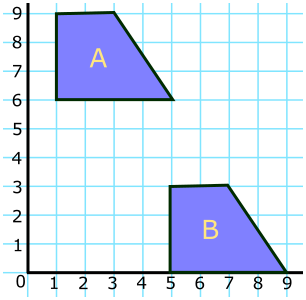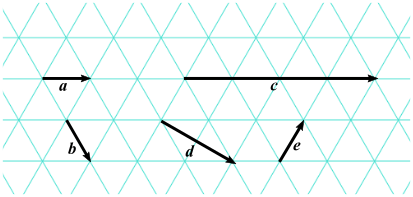# Exam-Style Questions on Transformations

## Problems on Transformations adapted from questions set in previous Mathematics exams.

### 1.

GCSE Higher

The shape A is drawn on the coordinate grid as shown below.Sally and Eddie each transform the shape A onto shape B.

• Sally uses a reflection in the line y = 7 followed by a rotation of 90o anticlockwise about the point (9,9).
• Eddie transforms shape A first with a reflection in the line $$y = x$$ followed by his favourite transformation.

(a) Draw and label shape B.

(b) Describe fully Eddie's favourite transformation.

### 2.

GCSE Higher

The diagram shows a red trapezium drawn on a grid.The trapezium is subjected to two transformations, one after the other.

One transformation is a reflection in the line $$y=x$$.

The other transformation is a reflection y-axis.

### 3.

GCSE Higher

(a) Shape $$A$$ is translated to shape $$B$$ using the vector $$\begin{pmatrix}m\\n\\ \end{pmatrix}$$. What are the values of $$m$$ and $$n$$?(b) Vectors $$a, b, c, d$$ and $$e$$ are drawn on an isometric grid. Write each of the vectors $$c, d$$ and $$e$$ in terms of $$a$$ and/or $$b$$.### 4.

GCSE Higher

Plot the following points in order then join them up in order to make an irregular hexagon.

$$(-3,-1), (-2,-2), (-1,-2), (0,-1), (-1,-4), (-2,-4)$$Enlarge the hexagon by a scale factor of $$2\frac12$$, centre (-3,-4).

The exam-style questions appearing on this site are based on those set in previous examinations (or sample assessment papers for future examinations) by the major examination boards. The wording, diagrams and figures used in these questions have been changed from the originals so that students can have fresh, relevant problem solving practice even if they have previously worked through the related exam paper.

The solutions to the questions on this website are only available to those who have a Transum Subscription.

Exam-Style Questions Main Page

Search for exam-style questions containing a particular word or phrase:

To search the entire Transum website use the search box in the grey area below.

Kausar Begum Khan, Kenya

Friday, January 26, 2018

"This is a fabulous website! I cannot thank you enough."

Tuesday, November 6, 2018

"Transum is so resourceful. The resources are so child-friendly that my students love its usage.It has a wide range of topics and caters to most of my needs. 'Thankyou" will be too small a word to express my gratitude to the content developers of this site."

Ahmed Jaja, Ghana

Thursday, November 22, 2018

"It was very useful for me.I recommend it to every math student.Thank you."

Tuesday, February 5, 2019

"A very great site.Nice questions."

Yuli, Woodmansterne

Tuesday, April 2, 2019

"This website is amazing it helps me with my maths to get good marks in my upcoming test. Thanks."

Do you have any comments? It is always useful to receive feedback and helps make this free resource even more useful for those learning Mathematics anywhere in the world. Click here to enter your comments.For All: